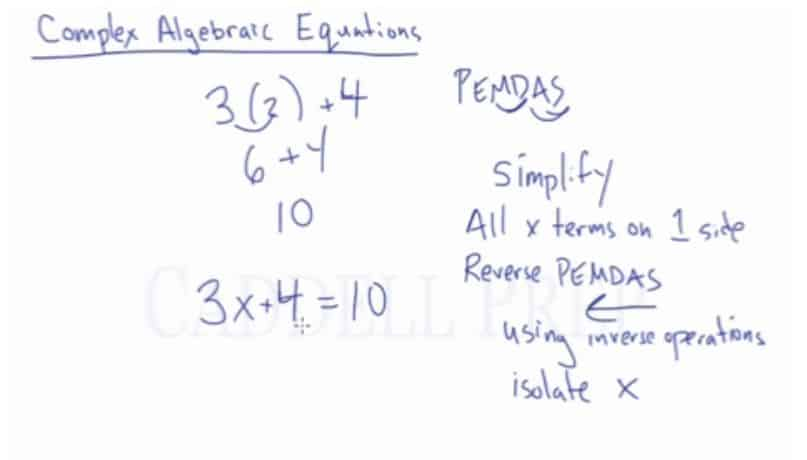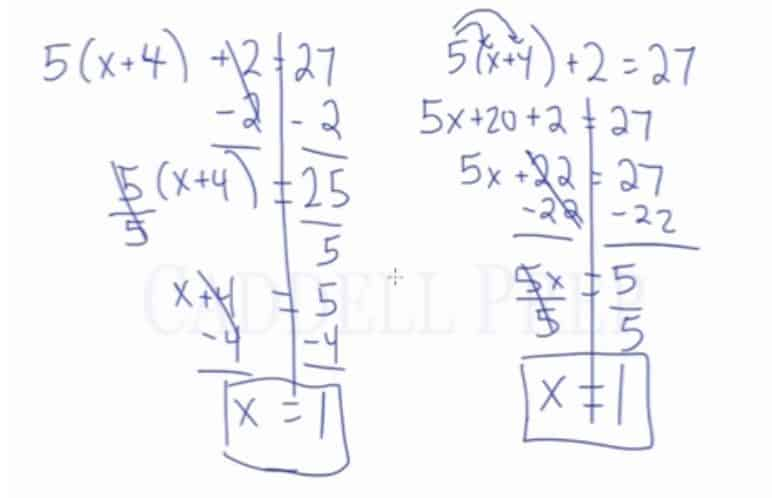In this video, we will be learning how to solve for x (or another variable) in complex algebraic equations using inverse operations. After you finish this lesson, view all of our Pre-Algebra and Algebra lessons and practice problems.

## Example of Solving a Multi-Step Algebraic Equation$5(x+4) +2 = 27$$5(x+4) +2 -2 = 27 -2 \leftarrow$ Subtract 2 from both sides$\frac {5(x+4)}{5} = \frac{25}{5} \leftarrow$ Divide by 5 on both sides$(x+4) -4 =5 -4\leftarrow$ Subtract 4 on both sides$x=1$

### Example 1$2(x+3) = 38$

First, distribute$2$ to the terms inside the parenthesis$2(x+3) = 38$$2x+6 = 38$

Then, subtract$6$ from both sides$2x+6-6 = 38-6$$2x=32 \leftarrow$ divide$2$ from both sides$\dfrac{2x}{2}=\dfrac{32}{2}$

Now, we have:$x=16$

### Example 2$3(4x-2)=42$

First, distribute$3$ to the terms inside the parenthesis$3(4x-2)=42$$12x-6=42$

Then, add$6$ from both sides$12x-6+6=42+6$$12x=48 \leftarrow$ divide$12$ from both sides$\dfrac{12x}{12}=\dfrac{48}{12}$

Now, we have:$x=4$

Another way of doing this problem:$5(x+4) +2 = 27 \leftarrow$ Distribute the 5 to the x and the 4$5x+20 +2 = 27 \leftarrow$ Simplify using addition$5x+22 -22 = 27 -22 \leftarrow$ Subtract 22 from both sides$\frac{5x}{5}=\frac{5}{5} \leftarrow$Divide by 5 on each side$x=1$

## Video-Lesson Transcript

Let’s get into solving complex algebraic equations. This involves more than one operation.

To review, order of operations or PEMDAS, we have$3(2) + 4$.

PEMDAS is an acronym which stands for
Parenthesis
Exponents
Multiplication
Division
Subtraction

Let’s evaluate$3(2) + 4$.

So$3 \times 2 + 4 = 6 + 4$ we’ll have$= 10$.

In solving algebraic equations, we don’t have all whole numbers. Instead, we have variables in it.

So, we may have$3x + 4 = 10$.

From our example, we already know that$x = 2$.

But let’s try solving this algebraically.

We just have to do few steps to solve this.
1. Simplify both sides of the equations, if possible.
2. If there’s$x$ terms on both sides, we have to get all the$x$ terms on one side. You can have it on the left side or on the right side, whichever you prefer.
3. Reverse PEMDAS. We’re going to do the order of operations backward using inverse operations.
4. Our goal is to isolate the variable$x$.Going back to$3x + 4 = 10$, let’s do the steps above.

1. Simplify – This is the simplest it can get.
2. All$x$ term on one side – There’s just one$x$ and it’s on the left.
3. Now, let’s do the reverse PEMDAS using inverse operations.

Let’s subtract$4$ on both sides of the equation.$3x + 4 - 4 = 10 - 4$

We’ll have$3x = 6$.

4. Isolate$x$

Here we have to divide both sides by$3$.$\dfrac{3x}{3} = \dfrac{6}{3}$

And we’ll have$x = 2$.

Let’s do another example.

We have$\dfrac{x}{4} - 3 = 1$

So, let’s start off by adding$3$ on both sides of the equation.$\dfrac{x}{4} - 3 + 3 = 1 + 3$

We’ll come up with$\dfrac{x}{4} = 4$

Then, we multiply$4$ on both sides.$\dfrac{x}{4} \times 4 = 4 \times4$

The answer is$x = 16$

Let’s have one more example. I’ll show you how to solve this using two different ways.

We have$5(x + 4) + 2 = 27$

First method of solving is this:

Let’s subtract$2$ on both sides of the equation.$5(x + 4) + 2 - 2 = 27 - 2$

We’ll have$5(x + 4) = 25$

Then, we have to divide both sides by$5$$\dfrac{5}{5} (x + 4) = \dfrac{25}{5}$

We’ll come up with$x + 4 = 5$

Then, to isolate$x$ we have to subtract$4$ on both sides$x + 4 - 4 = 5 - 4$

Our final answer is$x = 1$

So now let’s move on to the second method of solving the same equation.$5(x + 4) + 2 = 27$

The second method is to simplify the equation as much as we can.

Let’s start off by distributing$5$ into the equation in the parenthesis –$x + 4$

So let’s do it!$5 \times x$ and$5 \times 4$

We’ll have$5x + 20 + 2 = 27$

Now, we can combine like terms to simplify further$5x + 22 = 27$

Then we have to do the reverse PEMDAS.

Let’s subtract$22$ on both sides$5x + 22 - 22 = 27 - 22$

We’ll come up with$5x = 5$

Now, let’s do the inverse of multiplication which is division.

Divide both sides by$5$$\dfrac{5x}{5} = \dfrac{5}{5}$

Our final answer is$x = 1$

Both methods gave us the same answer$x = 1$.To sum up, no matter how complex our algebraic equation is, we can do reverse PEMDAS or inverse order of operations to isolate the$x$.